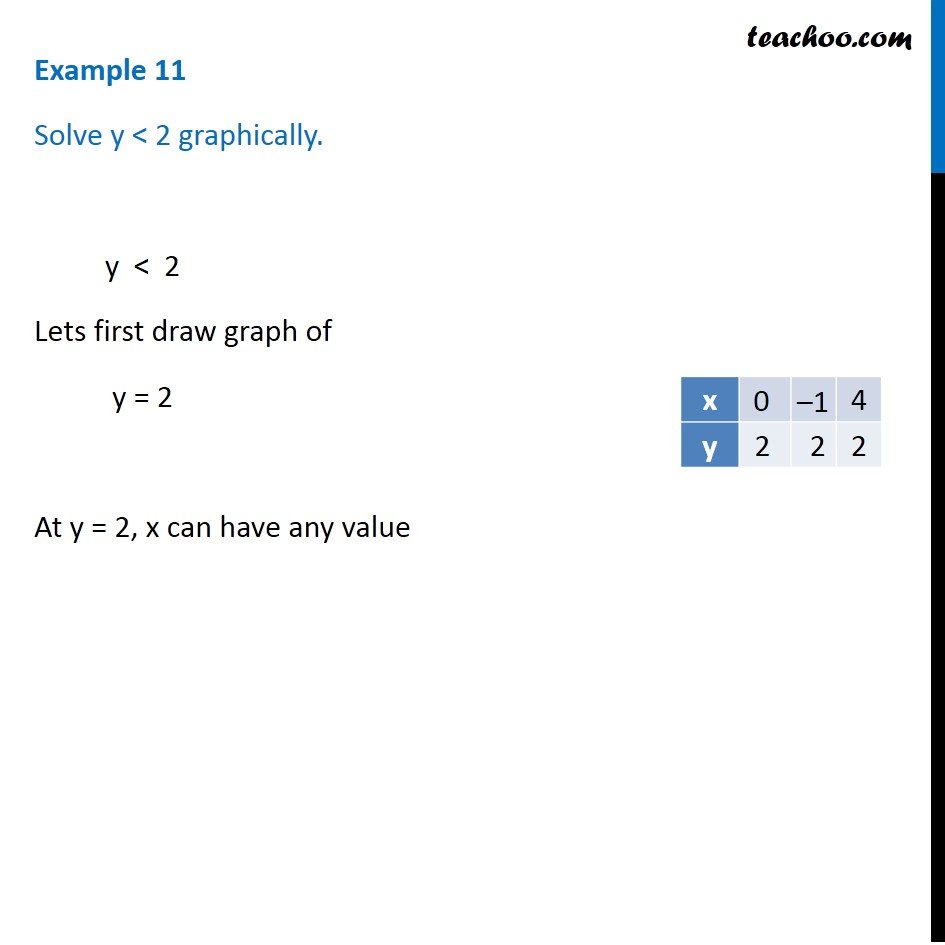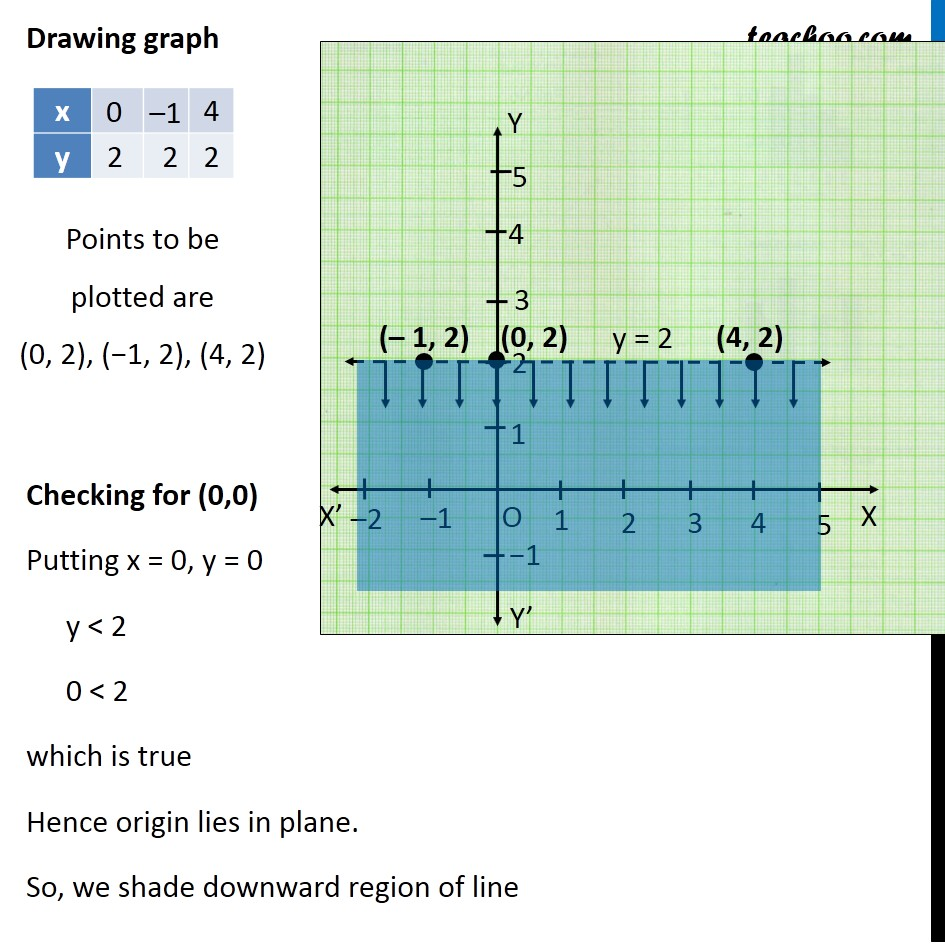Examples

Chapter 6 Class 11 Linear Inequalities
Serial order wiseIntroducing your new favourite teacher - Teachoo Black, at only ₹83 per month

### Transcript

Example 11 Solve y < 2 graphically. y < 2 Lets first draw graph of y = 2 At y = 2, x can have any value Points to be plotted are (0, 2), (−1, 2), (4, 2) Drawing graph Checking for (0,0) Putting x = 0, y = 0 y < 2 0 < 2 which is true Hence origin lies in plane. So, we shade downward region of line